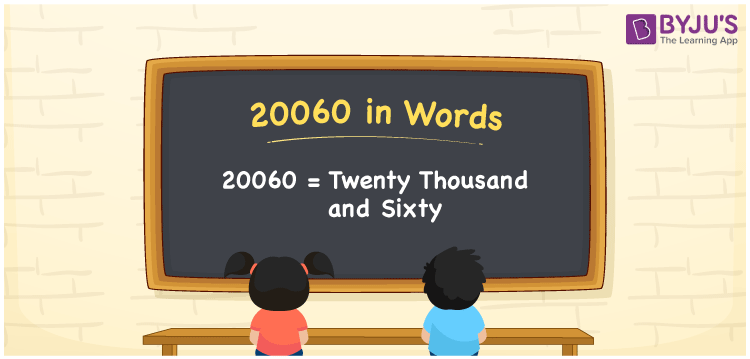# 20060 in Words

We can write 20060 in words as Twenty thousand and sixty. We can efficiently convert the number 20060 into words using a place value chart. Also, Twenty thousand and sixty is the number name of 20060. For example, your account is debited with Rs. 20060, then you can say, “My account is debited with Twenty thousand and sixty rupees”. Let’s learn how to write the number 20060 into words here in this article.

 20060 in words Twenty thousand and sixty Twenty thousand and sixty in Numbers 20060

## 20060 in English words

Generally, we write numbers in words using the English alphabet. Thus, we can spell 20060 in English as “Twenty thousand and sixty”.## How to Write 20060 in Words?

To derive the number 20060 into words, we need to create a place value chart with five columns as the number 20060 contains five digits, as shown below.

 Ten thousands Thousands Hundreds Tens Ones 2 0 0 6 0

Here, ones = 0, tens = 6, hundreds = 0, thousands = 0, ten thousands = 2

By expanding the above digits according to their place values, we get;

2 × Ten thousand + 0 × Thousand + 0 × Hundred + 6 × Ten + 0 × One

= 2 × 10000 + 6 × 10

= 20000 + 60

= Twenty thousand + Sixty

= Twenty thousand and sixty

Therefore, 20060 in words = Twenty thousand and sixty.

As we know, 20060 is a natural number that is the successor of 20059 and the predecessor of 20061.

20060 in words – Twenty thousand and sixty

Is 20060 an odd number? – No

Is 20060 an even number? – Yes

Is 20060 a perfect square number? – No

Is 20060 a perfect cube number? – No

Is 20060 a prime number? – No

Is 20060 a composite number? – Yes

## Frequently Asked Questions on 20060 in Words

Q1

### How do you write 20060 in words?

We generally write the number 20060 in English words as Twenty thousand and sixty.
Q2

### How to express Rs. 20060 in words on a cheque?

On a cheque, we generally express Rs. 20060 in words as “Twenty thousand and sixty rupees only”.
Q3

### Find the value of 20060 – 17000. Write the answer in words.

20060 – 17000 = 3060 Therefore, the value of 20060 – 17000, i.e., 3060 in words is Three thousand and sixty.